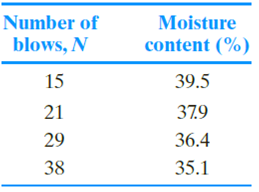Chapter 4, Problem 4.6PPrinciples of Geotechnical Enginee...

9th Edition
Braja M. Das + 1 other
ISBN: 9781305970939

Solutions

Chapter
SectionPrinciples of Geotechnical Enginee...

9th Edition
Braja M. Das + 1 other
ISBN: 9781305970939
Textbook Problem

Refer to the soil in Problem 4.5. Using the Casagrande plasticity chart, graphically estimate the shrinkage limit of the soil as shown in Figure 4.22.4.5 The following data were obtained by conducting liquid limit and plastic limit tests on a soil collected from the site.Liquid limit tests:Plastic limit test: PL = 19.3%a. Draw the flow curve and determine the liquid limit.b. Using the Casagrande plasticity chart (Figure 4.21), determine the soil type.

To determine

Draw the Casagrande plasticity chart to estimate the shrinkage limit of the soil.

Explanation

Given information:

The plastic limit of the soil PL is 19.3 %.

Calculation:

Draw the graph between the number of flows and moisture content as in Figure 1.

Refer Figure 1.

The liquid limit value for the 25 number of blows is 37.05 %.

Determine the plasticity index of the soil PI using the relation.

PI=LLPL

Substitute 37.05 % for LL and 19.3 % for PL.

PI=37.0519.3=17

Still sussing out bartleby?

Check out a sample textbook solution.

See a sample solution

The Solution to Your Study Problems

Bartleby provides explanations to thousands of textbook problems written by our experts, many with advanced degrees!

Get Started

What is joint application design?

Management Of Information Security

What e agile methods? Are they better than traditional methods? Why or why not?

Systems Analysis and Design (Shelly Cashman Series) (MindTap Course List)

Which of the following is not an example of an output device? a. scanner b. printer c. display d. speaker

Enhanced Discovering Computers 2017 (Shelly Cashman Series) (MindTap Course List)